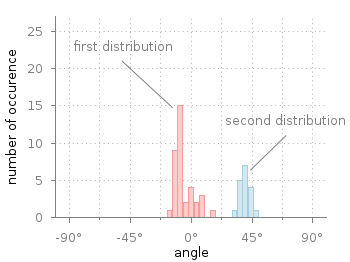# Gnuplotting

Create scientific plots using gnuplot

April 16th, 2014 | 17 Comments

Gnuplot comes with the possibility of plotting histograms, but this requires that the data in the individual bins was already calculated. Here, we start with an one dimensional set of data that we want to count and plot as an histogram, similar to the `hist()` function we find in Octave.Fig. 1 Two different distributions of measured angles. (code to produce this figure, hist.fct, data)

In Fig. 1 you see two different distributions of measured angles. They were both given as one dimensional data and plotted with a defined macro that is doing the histogram calculation. The macro is defined in an additional file `hist.fct` and loaded before the plotting command.

```binwidth = 4
binstart = -100
plot 'histogram.txt' i 0 @hist ls 1,\
''              i 1 @hist ls 2
```

The content of `hist.fct`, including the definition of `@hist` looks like this

```# set width of single bins in histogram
set boxwidth 0.9*binwidth
# set fill style of bins
set style fill solid 0.5
# define macro for plotting the histogram
hist = 'u (binwidth*(floor((\$1-binstart)/binwidth)+0.5)+binstart):(1.0) smooth freq w boxes'
```

For a detailed discussion on why `@hist` calculates a histogram you should have a look at this discussion and the documentation about the smooth freq which basically counts points with the same x-value. The other settings in the file define the width of a single bin plotted as a box and its fill style.Fig. 2 Two different distributions of measured angles. The bins of the histograms are shifted to be centered around 0°. (code to produce this figure, hist.fct, data)

It is important that the two values `binwidth` and `binstart` are defined before loading the `hist.fct` file. These define the width of the single bins and at what position the left border of a single bin should be positioned. For example, let us assume that we want to have the bins centered around 0° as shown in Fig. 2. This can be achieved by settings the binstart to half the binwidth:

```binwidth = 4
binstart = -2